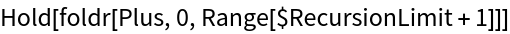Function Repository Resource:

FoldRight

Compute a right-associated fold

Contributed by: Richard Hennigan (Wolfram Research)
 ResourceFunction["FoldRight"][f,x,list] gives the right-associated fold of f over the given arguments. ResourceFunction["FoldRight"][f,list] is equivalent to ResourceFunction["FoldRight"][f,Last[list],Most[list]]. ResourceFunction["FoldRight"][f] represents an operator form of ResourceFunction["FoldRight"] that can be applied to expressions.

Details and Options

ResourceFunction["FoldRight"] is often called foldr.
ResourceFunction["FoldRight"][f,x,list] is effectively equivalent to Fold[f[#2,#1]&,x,Reverse[list]].
You can use Throw to exit from ResourceFunction["FoldRight"] before it is finished.
ResourceFunction["FoldRight"][f][list] is equivalent to ResourceFunction["FoldRight"][f,list].
ResourceFunction["FoldRight"][f][x,list] is equivalent to ResourceFunction["FoldRight"][f,x,list].

Examples

Basic Examples (6)

Perform a right fold on a list:

 In:=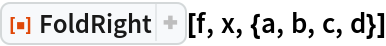Out=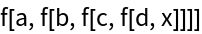Perform another right fold:

 In:=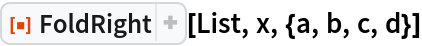Out=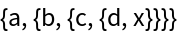Use a right fold to form a power tower:

 In:=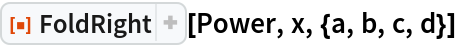Out=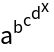Use the last element of the list as the starting value:

 In:=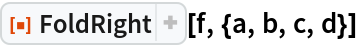Out=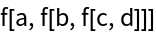Use the operator form of FoldRight on one argument:

 In:=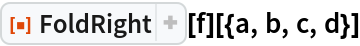Out=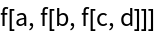Use the operator form of FoldRight on two arguments:

 In:=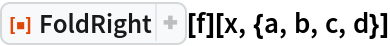Out=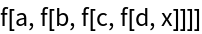Scope (1)

Perform a chain of cross products:

 In:=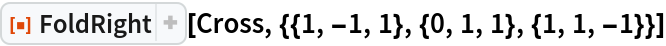Out=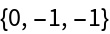Generalizations and Extensions (2)

The head need not be List:

 In:=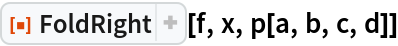Out=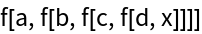Fold to the left:

 In:=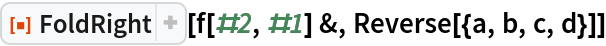Out=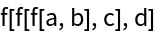Or equivalently:

 In:=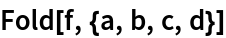Out=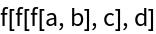Applications (5)

Form an alternating sum:

 In:=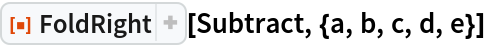Out=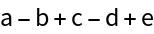Form a continued fraction:

 In:=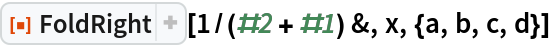Out=Form a binary tree:

 In:=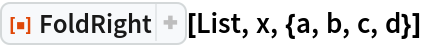Out=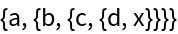FoldRight can be used to perform composition:

 In:=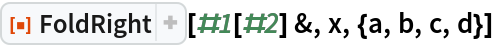Out=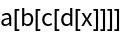Or equivalently:

 In:=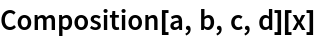Out=Similarly, Fold can be used to form a right composition:

 In:=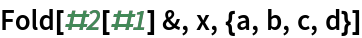Out=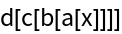In:=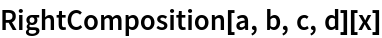Out=Form a composition of subvalues:

 In:=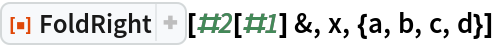Out=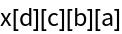Properties and Relations (5)

Folding with an empty list does not apply the function at all:

 In:=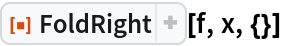Out=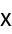Functions that ignore their first argument give the same result as Nest:

 In:=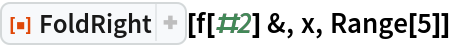Out=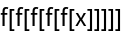In:=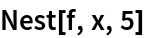Out=Compare FoldRight to Fold structurally:

 In:=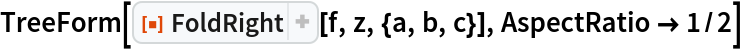Out=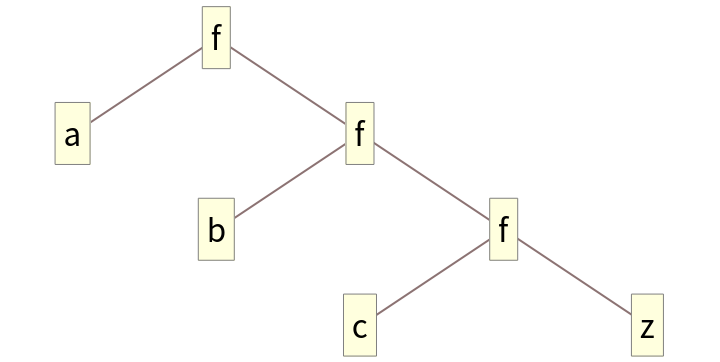In:=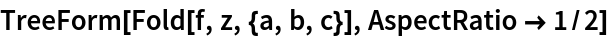Out=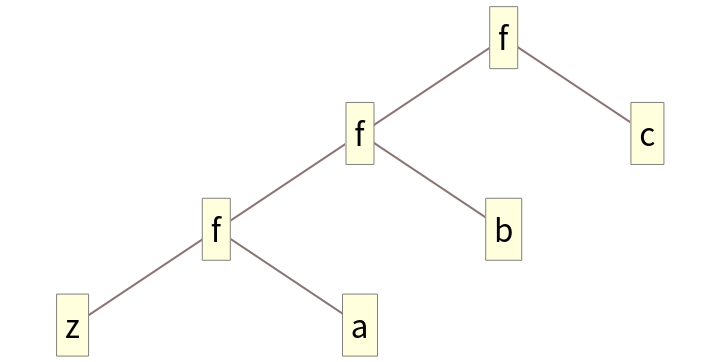The order of arguments passed to f are reversed compared to Fold:

 In:=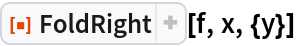Out=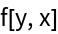In:=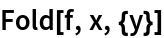Out=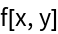FoldRight takes elements starting on the right of the given list:

 In:=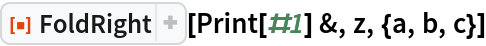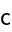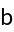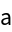Compare to Fold:

 In:=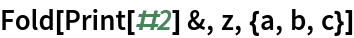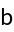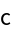Possible Issues (2)

An empty list cannot be folded:

 In:=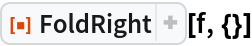Out=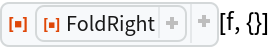Elements are taken in reverse order:

 In:=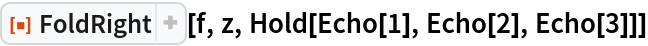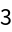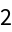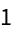Out=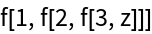Compare to a typical recursive definition of foldr:

 In:=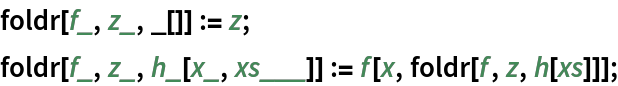In:=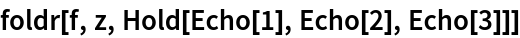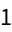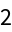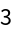Out=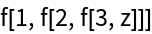This implementation allows FoldRight to be tail recursive, which is far more efficient with respect to stack size:

 In:=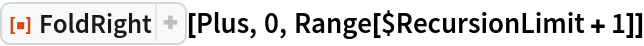Out=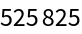Without tail recursion, the stack size would grow with respect to the size of the list:

 In:=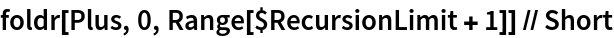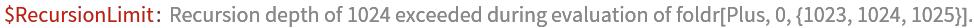Out=